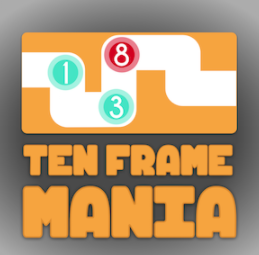# Ten Frame Mania: A Game’s Worth of Practice

Knowledge Constructor and Computational Thinker### Overview​

In this online game, Ten Frame Mania by Greg Tang, students make a given number in a digital ten frame to win the game for numbers up to 20. Thinking about numbers using frames of 10 can be a helpful way to learn basic number facts.
In addition, students can use benchmark numbers to count up or down to their desired number using the fill feature of the game.

Students will:

• Be able to make a given number from 1 to 20 using digital ten frames.
• Be able to use a digital fill feature to count up or down from benchmark numbers like 5, 10, 15, and 20 to a given number.

Vocabulary Words:

1. Ten Frame: A ten frame is a 2-by-5 rectangular container used to hold counters that illustrate numbers.
2. Benchmark Number: A benchmark number is a number by which other numbers can be easily estimated or compared.

To prepare for this lesson:

• To prepare for this task, the teacher should:

• Have at least one device per two students that has internet access. This activity will work best if there is a one-to-one device to child ratio.

• Ensure the Ten Frame Mania website is not blocked.

• Watch the tutorial video and/or play the tutorial video for students in a whole-class setting.

See Accommodations Page and Charts on the 21things4students.net site in the Teacher Resources.

Directions for this activity:

1. Watch the tutorial as a whole class or direct students to the tutorial at: https://www.gregtangmath.com/tenframemania.

2. Have students play the Ten Frame Mania game.

Different options for assessing the students:

• Observations
• Check for understanding
• To assess a students’ mastery over these concepts, teachers could create an online quiz/game that asked questions like the following:
1. Which tens-frame shows ____?  (Insert any given number in the blank from 1 - 20.) Given a filled ten frame. What number is represented by the ten frame(s)?
2. What benchmark number is most helpful to get us to ____?
• Students could complete a math reflection on any of the following questions?
1. When was it most helpful to use the fill feature on the game?
2. Do you have to always use a filled ten frame when you have numbers over 10?
3. When does it make the most sense to use the fill features versus counting each counter individually?
4. What helped you to become a better player in the game?

MITECS: Michigan adopted the "ISTE Standards for Students" called MITECS (Michigan Integrated Technology Competencies for Students) in 2018.

Knowledge Constructor
3d. Students build knowledge by actively exploring real-world issues and problems, developing ideas and theories and pursuing answers and solutions.

Computational Thinker
5c. Students break problems into component parts, extract key information, and develop descriptive models to understand complex systems or facilitate problem-solving.

Browser: Chrome, Safari, Firefox, Edge, ALL

Websites:
Ten Frame Mania Website

##### CONTENT AREA RESOURCES

Numbers and Operations in Base Ten

Kindergarten
Work with numbers 11-19 to gain foundations for place value.

CCSS.MATH.CONTENT.K.NBT.A.1
Compose and decompose numbers from 11 to 19 into ten ones and some further ones, e.g., by using objects or drawings, and record each composition or decomposition by a drawing or equation (such as 18 = 10 + 8); understand that these numbers are composed of ten ones and one, two, three, four, five, six, seven, eight, or nine ones.

Extend the counting sequence.

CCSS.MATH.CONTENT.1.NBT.A.1

Count to 120, starting at any number less than 120. In this range, read and write numerals and represent a number of objects with a written numeral.

Understand place value.

CCSS.MATH.CONTENT.1.NBT.B.2

Understand that the two digits of a two-digit number represent amounts of tens and ones. Understand the following as special cases:

CCSS.MATH.CONTENT.1.NBT.B.2.A

10 can be thought of as a bundle of ten ones — called a "ten."

CCSS.MATH.CONTENT.1.NBT.B.2.B

The numbers from 11 to 19 are composed of a ten and one, two, three, four, five, six, seven, eight, or nine ones.

Counting and Cardinality
Kindergarten
Know number names and the count sequence.

CCSS.MATH.CONTENT.K.CC.A.1

Count to 100 by ones and by tens.

CCSS.MATH.CONTENT.K.CC.A.2

Count forward beginning from a given number within the known sequence (instead of having to begin at 1).

CCSS.MATH.CONTENT.K.CC.A.3
Write numbers from 0 to 20. Represent a number of objects with a written numeral 0-20 (with 0 representing a count of no objects).

Count to tell the number of objects.

CCSS.MATH.CONTENT.K.CC.B.4
Understand the relationship between numbers and quantities; connect counting to cardinality.

CCSS.MATH.CONTENT.K.CC.B.4.A
When counting objects, say the number names in the standard order, pairing each object with one and only one number name and each number name with one and only one object.

CCSS.MATH.CONTENT.K.CC.B.4.B
Understand that the last number name said tells the number of objects counted. The number of objects is the same regardless of their arrangement or the order in which they were counted.

CCSS.MATH.CONTENT.K.CC.B.4.C
Understand that each successive number name refers to a quantity that is one larger.

CCSS.MATH.CONTENT.K.CC.B.5
Count to answer "how many?" questions about as many as 20 things arranged in a line, a rectangular array, or a circle, or as many as 10 things in a scattered configuration; given a number from 1-20, count out that many objects.

CREDITS
This task card was created by Jamila Kai Mathis, Shelby Twp, MI, March 2020.